Скачать презентацию Randomized matrix algorithms and their applications Petros Drineas

e0642c6280a4b8269bbecafca54b984f.ppt

• Количество слайдов: 38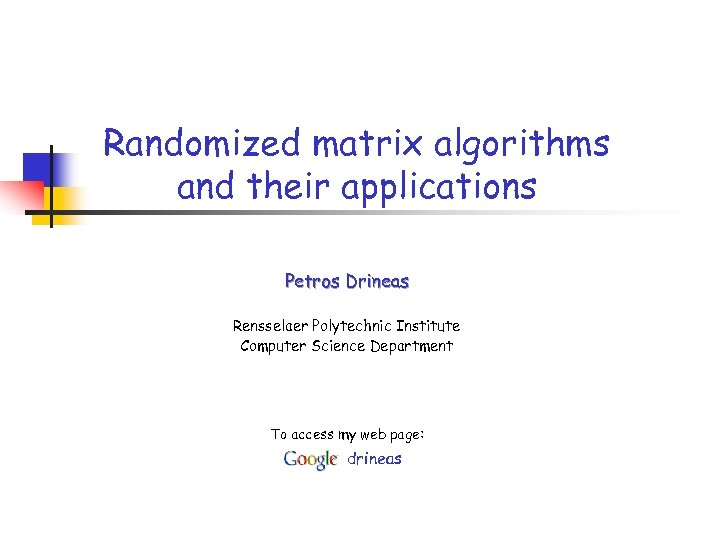Randomized matrix algorithms and their applications Petros Drineas Rensselaer Polytechnic Institute Computer Science Department To access my web page: drineas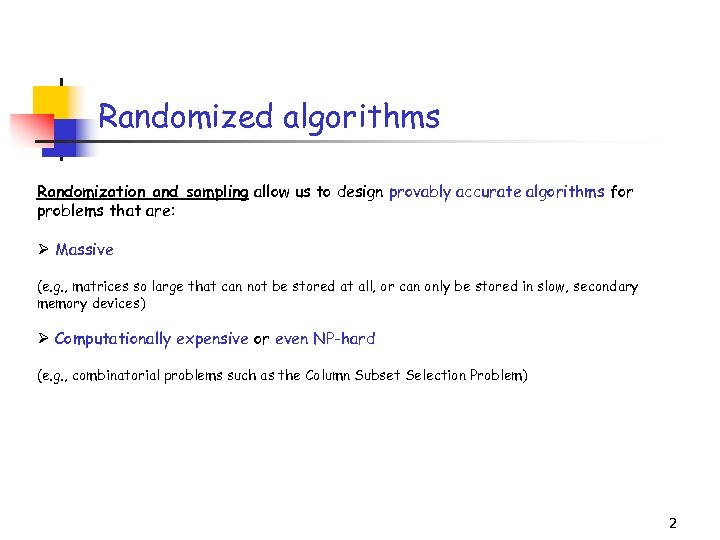Randomized algorithms Randomization and sampling allow us to design provably accurate algorithms for problems that are: Ø Massive (e. g. , matrices so large that can not be stored at all, or can only be stored in slow, secondary memory devices) Ø Computationally expensive or even NP-hard (e. g. , combinatorial problems such as the Column Subset Selection Problem) 2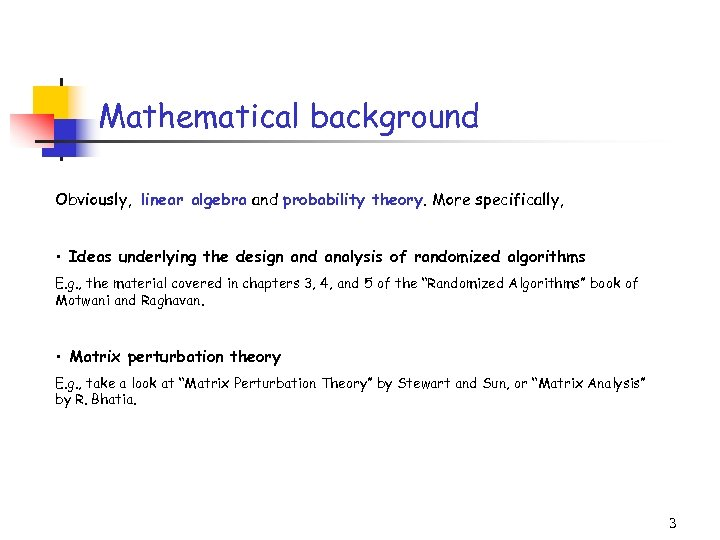Mathematical background Obviously, linear algebra and probability theory. More specifically, • Ideas underlying the design and analysis of randomized algorithms E. g. , the material covered in chapters 3, 4, and 5 of the “Randomized Algorithms” book of Motwani and Raghavan. • Matrix perturbation theory E. g. , take a look at “Matrix Perturbation Theory” by Stewart and Sun, or “Matrix Analysis” by R. Bhatia. 3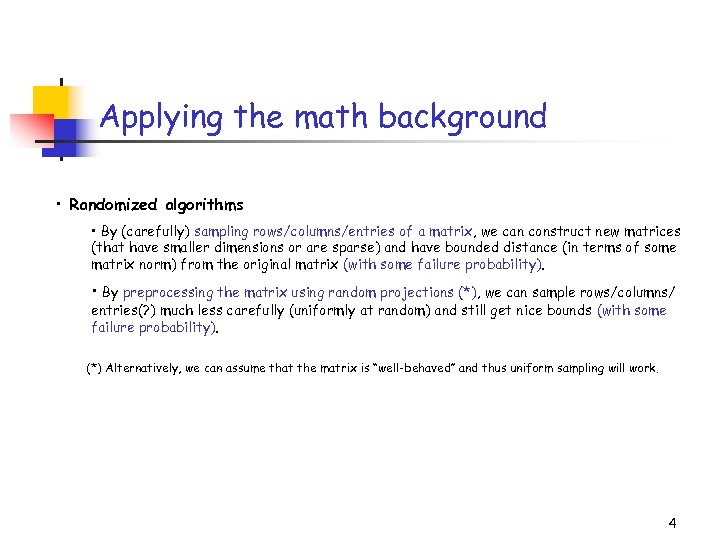Applying the math background • Randomized algorithms • By (carefully) sampling rows/columns/entries of a matrix, we can construct new matrices (that have smaller dimensions or are sparse) and have bounded distance (in terms of some matrix norm) from the original matrix (with some failure probability). • By preprocessing the matrix using random projections (*), we can sample rows/columns/ entries(? ) much less carefully (uniformly at random) and still get nice bounds (with some failure probability). (*) Alternatively, we can assume that the matrix is “well-behaved” and thus uniform sampling will work. 4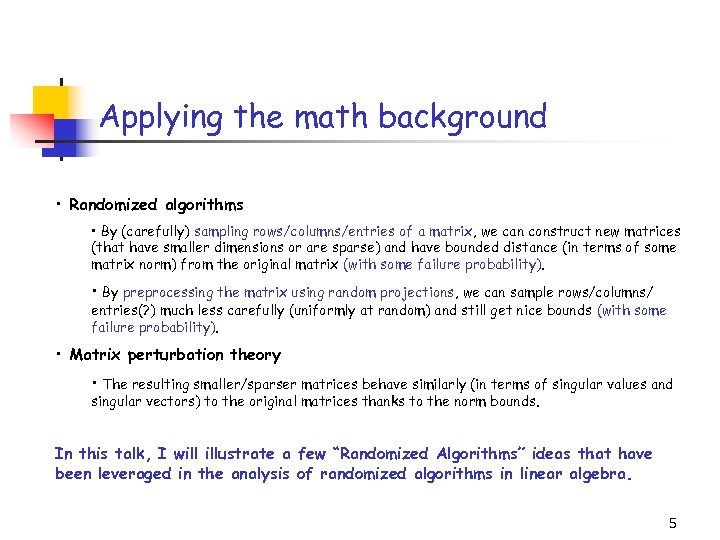Applying the math background • Randomized algorithms • By (carefully) sampling rows/columns/entries of a matrix, we can construct new matrices (that have smaller dimensions or are sparse) and have bounded distance (in terms of some matrix norm) from the original matrix (with some failure probability). • By preprocessing the matrix using random projections, we can sample rows/columns/ entries(? ) much less carefully (uniformly at random) and still get nice bounds (with some failure probability). • Matrix perturbation theory • The resulting smaller/sparser matrices behave similarly (in terms of singular values and singular vectors) to the original matrices thanks to the norm bounds. In this talk, I will illustrate a few “Randomized Algorithms” ideas that have been leveraged in the analysis of randomized algorithms in linear algebra. 5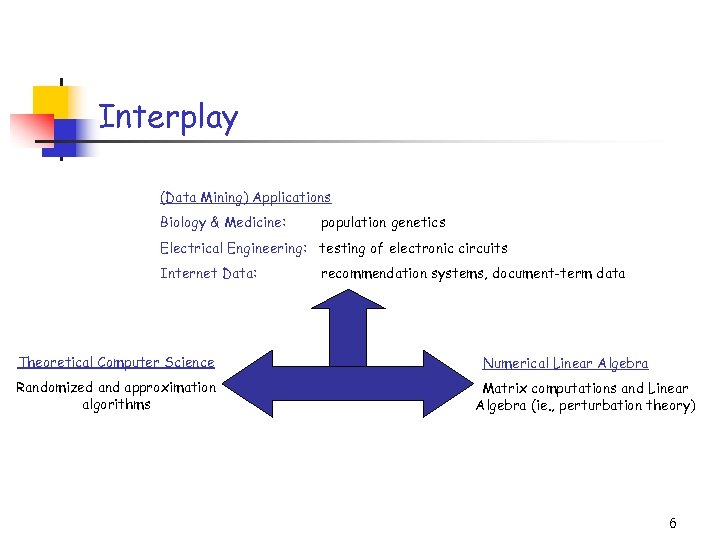Interplay (Data Mining) Applications Biology & Medicine: population genetics Electrical Engineering: testing of electronic circuits Internet Data: Theoretical Computer Science Randomized and approximation algorithms recommendation systems, document-term data Numerical Linear Algebra Matrix computations and Linear Algebra (ie. , perturbation theory) 6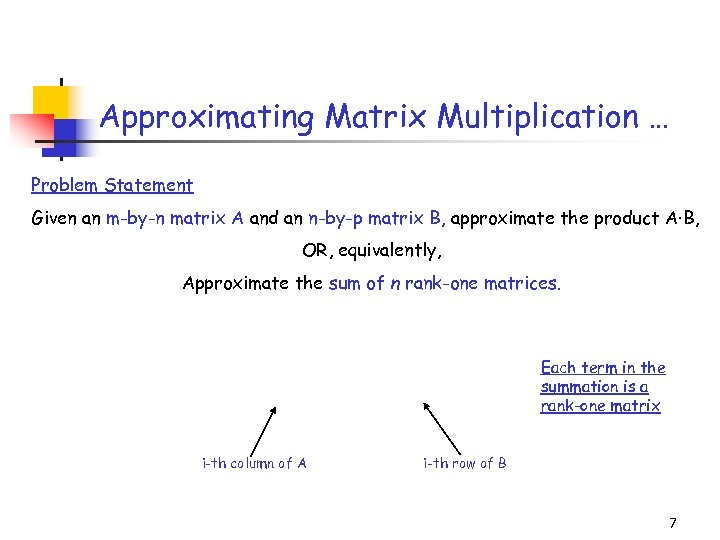Approximating Matrix Multiplication … Problem Statement Given an m-by-n matrix A and an n-by-p matrix B, approximate the product A·B, OR, equivalently, Approximate the sum of n rank-one matrices. Each term in the summation is a rank-one matrix i-th column of A i-th row of B 7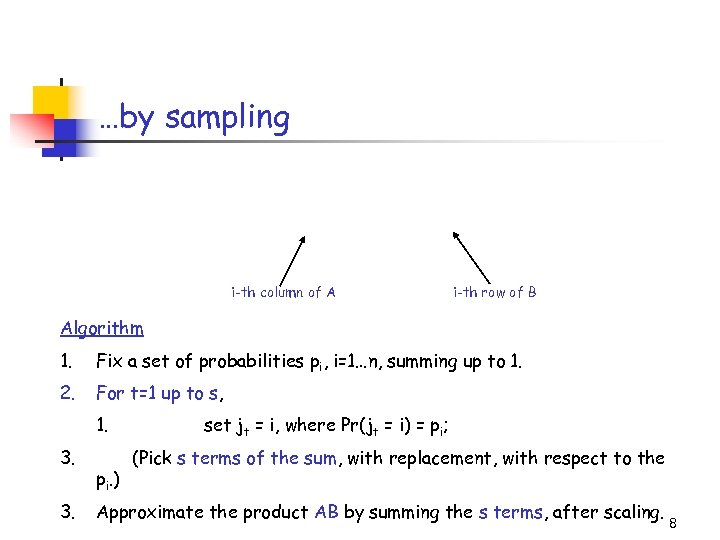…by sampling i-th column of A i-th row of B Algorithm 1. Fix a set of probabilities pi, i=1…n, summing up to 1. 2. For t=1 up to s, 1. 3. 3. pi. ) set jt = i, where Pr(jt = i) = pi; (Pick s terms of the sum, with replacement, with respect to the Approximate the product AB by summing the s terms, after scaling. 8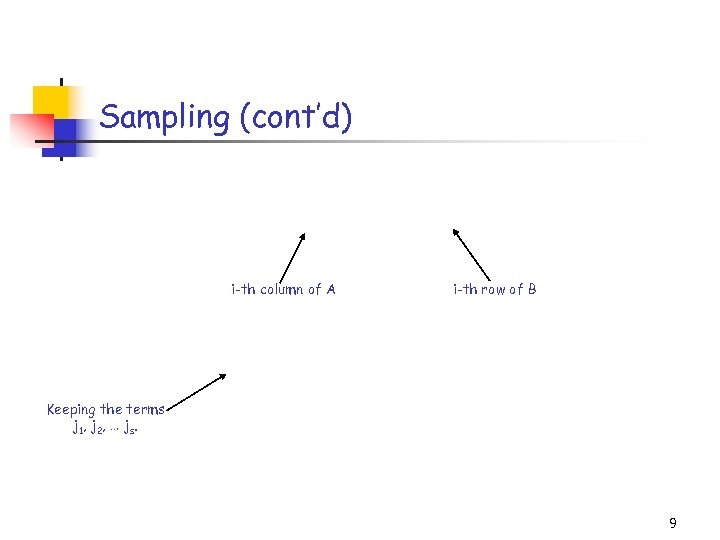Sampling (cont’d) i-th column of A i-th row of B Keeping the terms j 1, j 2, … js. 9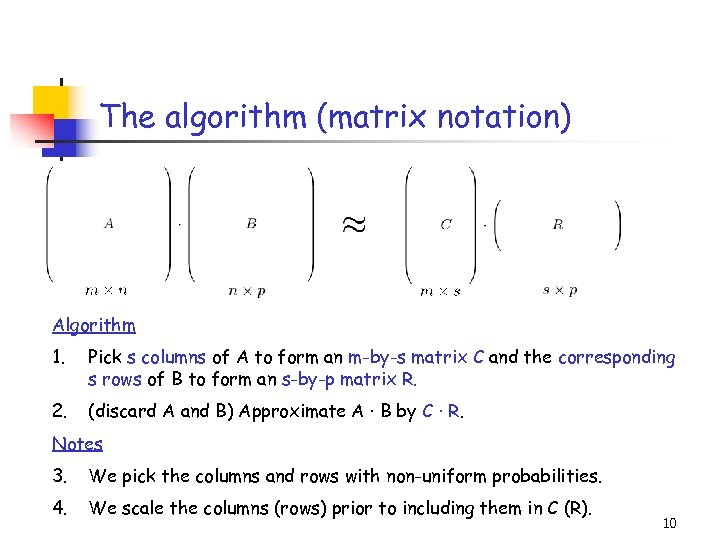The algorithm (matrix notation) Algorithm 1. Pick s columns of A to form an m-by-s matrix C and the corresponding s rows of B to form an s-by-p matrix R. 2. (discard A and B) Approximate A · B by C · R. Notes 3. We pick the columns and rows with non-uniform probabilities. 4. We scale the columns (rows) prior to including them in C (R). 10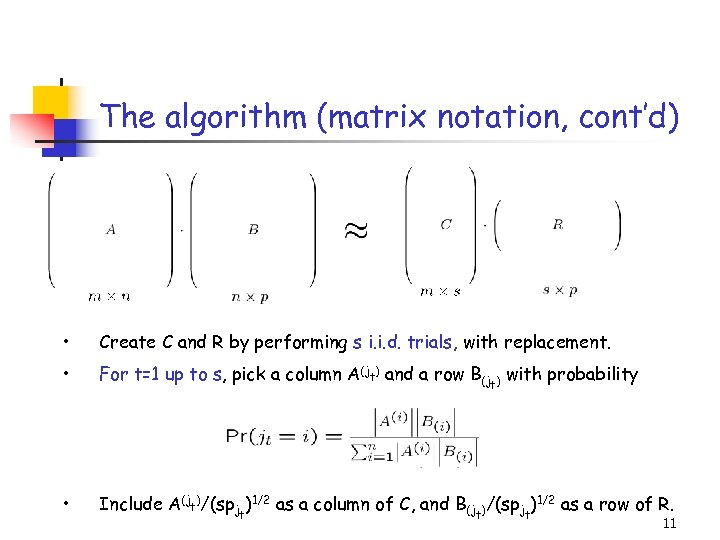The algorithm (matrix notation, cont’d) • Create C and R by performing s i. i. d. trials, with replacement. • For t=1 up to s, pick a column A(jt) and a row B(jt) with probability • Include A(jt)/(spjt)1/2 as a column of C, and B(jt)/(spjt)1/2 as a row of R. 11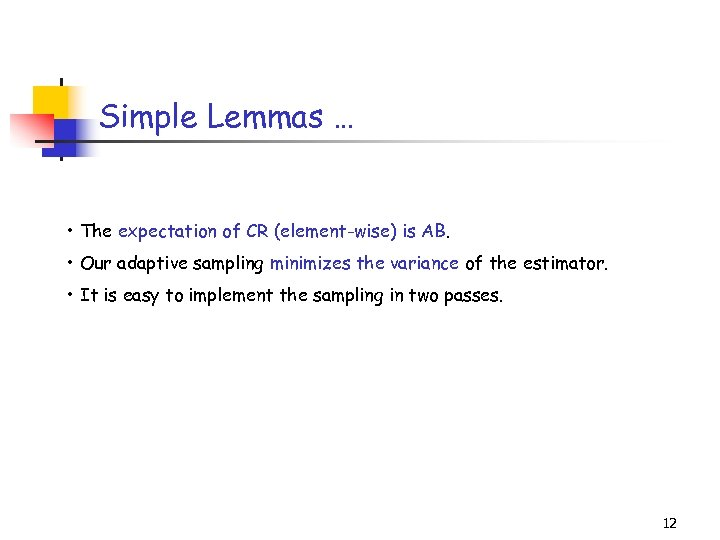Simple Lemmas … • The expectation of CR (element-wise) is AB. • Our adaptive sampling minimizes the variance of the estimator. • It is easy to implement the sampling in two passes. 12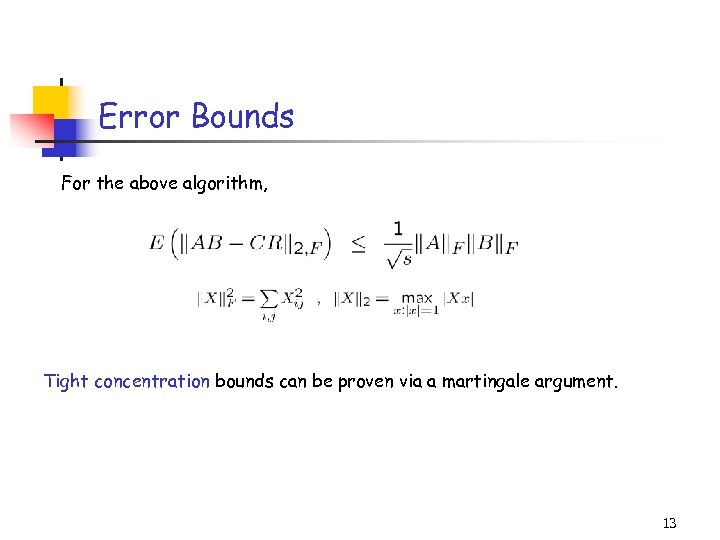Error Bounds For the above algorithm, Tight concentration bounds can be proven via a martingale argument. 13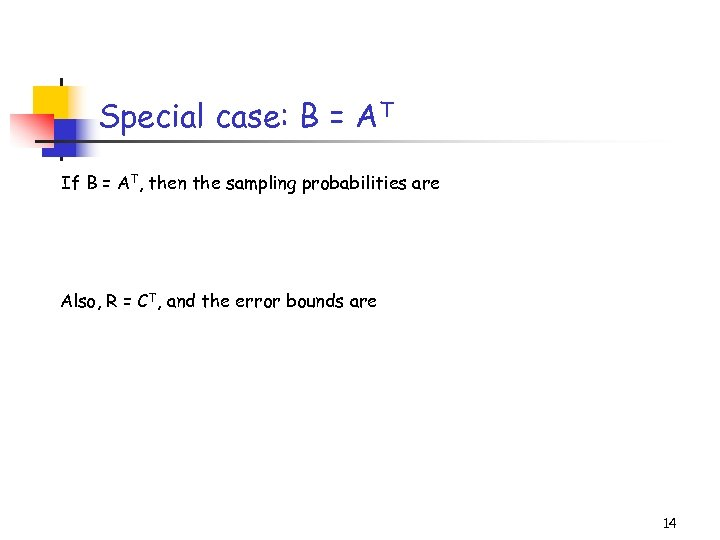Special case: B = AT If B = AT, then the sampling probabilities are Also, R = CT, and the error bounds are 14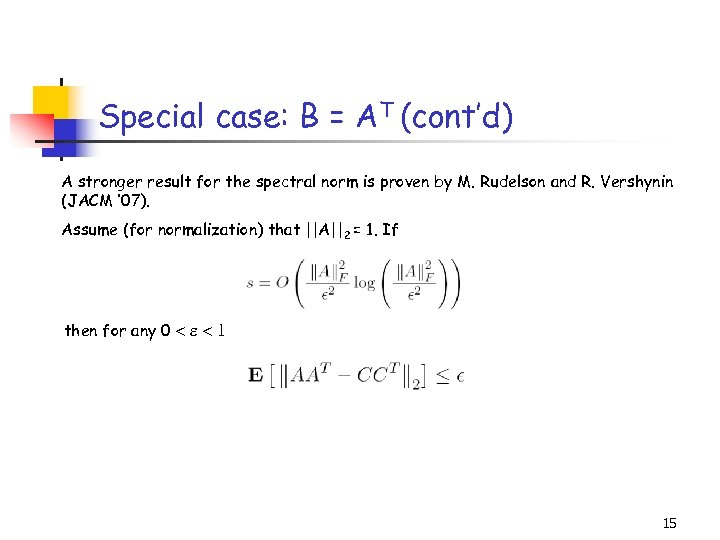Special case: B = AT (cont’d) A stronger result for the spectral norm is proven by M. Rudelson and R. Vershynin (JACM ’ 07). Assume (for normalization) that ||A||2 = 1. If then for any 0 < < 1 15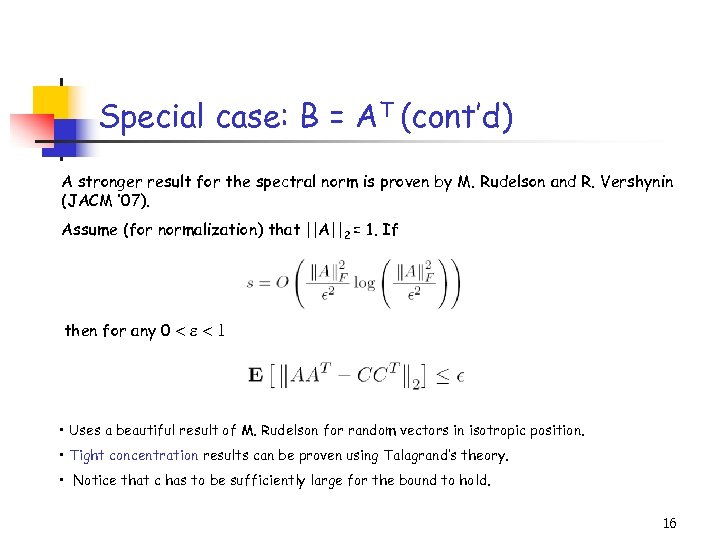Special case: B = AT (cont’d) A stronger result for the spectral norm is proven by M. Rudelson and R. Vershynin (JACM ’ 07). Assume (for normalization) that ||A||2 = 1. If then for any 0 < < 1 • Uses a beautiful result of M. Rudelson for random vectors in isotropic position. • Tight concentration results can be proven using Talagrand’s theory. • Notice that c has to be sufficiently large for the bound to hold. 16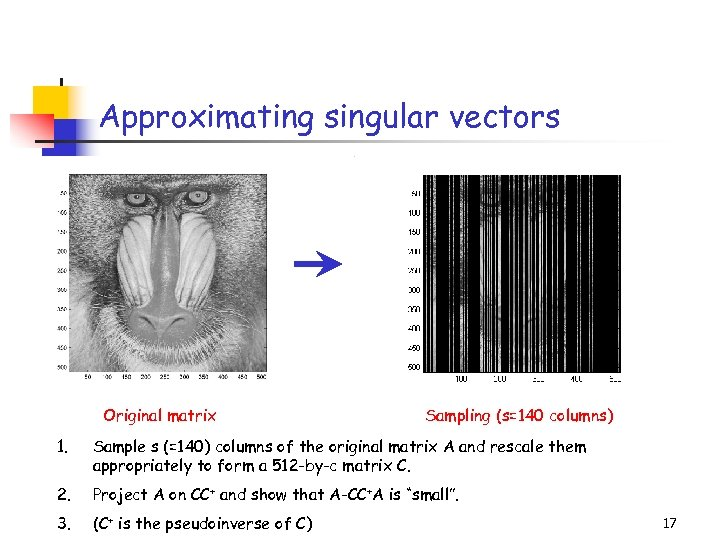Approximating singular vectors Title: C: PetrosImage Processingbaboondet. eps Creator: MATLAB, The Mathworks, Inc. Preview: This EPS picture was not saved with a preview included in it. Comment: This EPS picture will print to a Post. Script printer, but not to other types of printers. Original matrix Sampling (s=140 columns) 1. Sample s (=140) columns of the original matrix A and rescale them appropriately to form a 512 -by-c matrix C. 2. Project A on CC+ and show that A-CC+A is “small”. 3. (C+ is the pseudoinverse of C) 17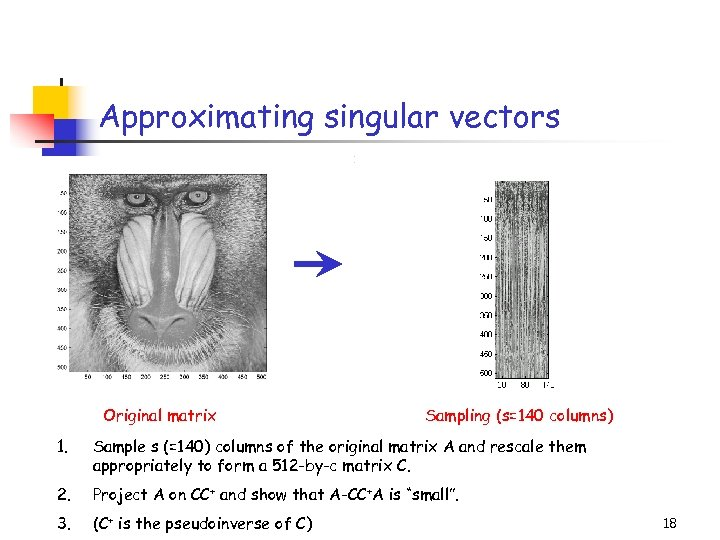Approximating singular vectors Title: C: PetrosImage Processingbaboondet. eps Creator: MATLAB, The Mathworks, Inc. Preview: This EPS picture was not saved with a preview included in it. Comment: This EPS picture will print to a Post. Script printer, but not to other types of printers. Original matrix Sampling (s=140 columns) 1. Sample s (=140) columns of the original matrix A and rescale them appropriately to form a 512 -by-c matrix C. 2. Project A on CC+ and show that A-CC+A is “small”. 3. (C+ is the pseudoinverse of C) 18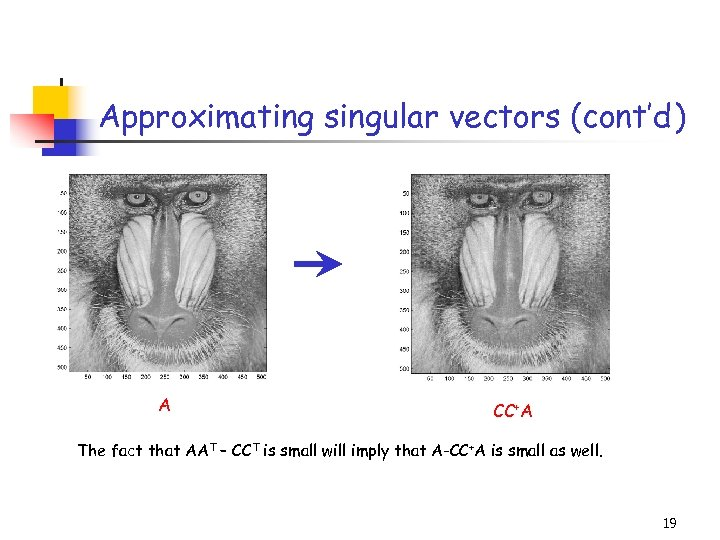Approximating singular vectors (cont’d) Title: C: PetrosImage Processingbaboondet. eps Creator: MATLAB, The Mathworks, Inc. Preview: This EPS picture was not saved with a preview included in it. Comment: This EPS picture will print to a Post. Script printer, but not to other types of printers. A CC+A The fact that AAT – CCT is small will imply that A-CC+A is small as well. 19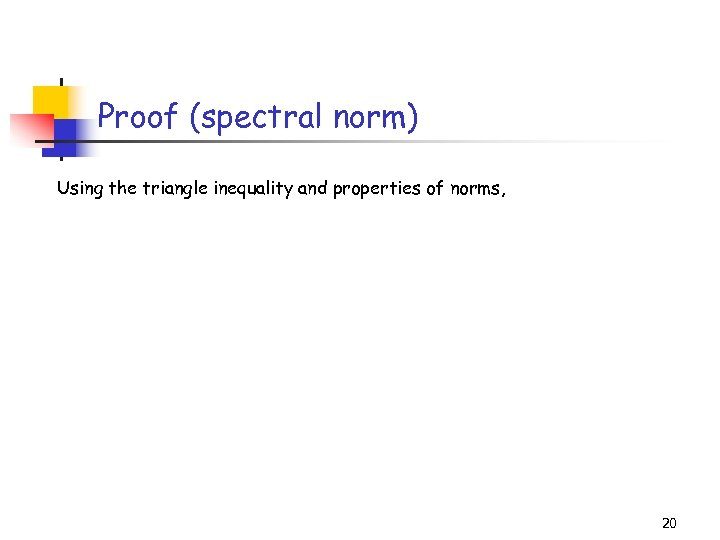Proof (spectral norm) Using the triangle inequality and properties of norms, 20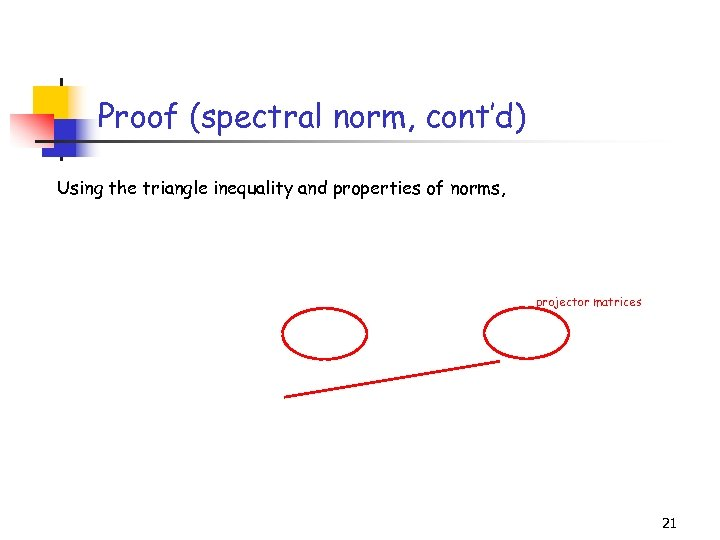Proof (spectral norm, cont’d) Using the triangle inequality and properties of norms, projector matrices 21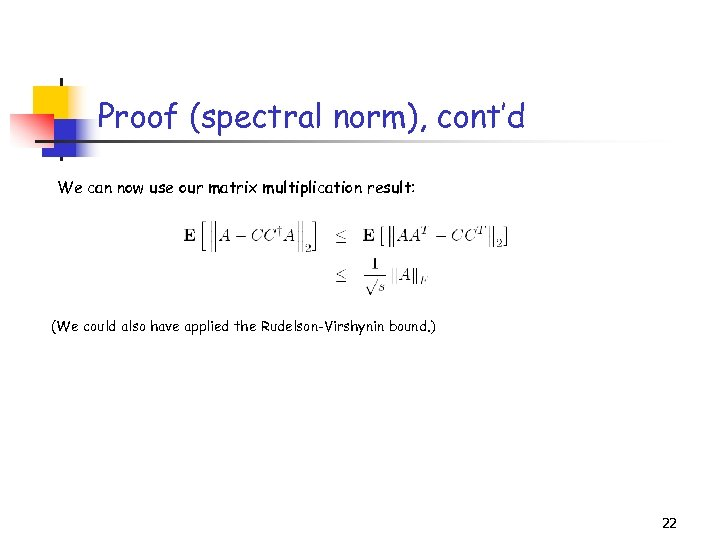Proof (spectral norm), cont’d We can now use our matrix multiplication result: (We could also have applied the Rudelson-Virshynin bound. ) 22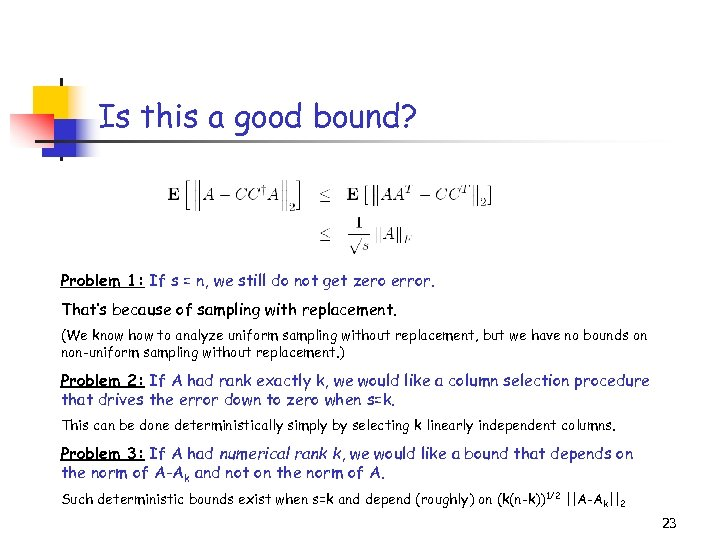Is this a good bound? Problem 1: If s = n, we still do not get zero error. That’s because of sampling with replacement. (We know how to analyze uniform sampling without replacement, but we have no bounds on non-uniform sampling without replacement. ) Problem 2: If A had rank exactly k, we would like a column selection procedure that drives the error down to zero when s=k. This can be done deterministically simply by selecting k linearly independent columns. Problem 3: If A had numerical rank k, we would like a bound that depends on the norm of A-Ak and not on the norm of A. Such deterministic bounds exist when s=k and depend (roughly) on (k(n-k)) 1/2 ||A-Ak||2 23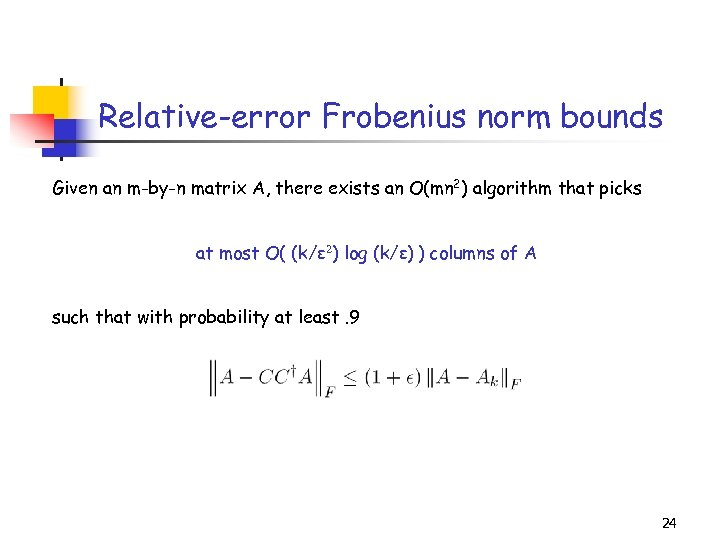Relative-error Frobenius norm bounds Given an m-by-n matrix A, there exists an O(mn 2) algorithm that picks at most O( (k/ε 2) log (k/ε) ) columns of A such that with probability at least. 9 24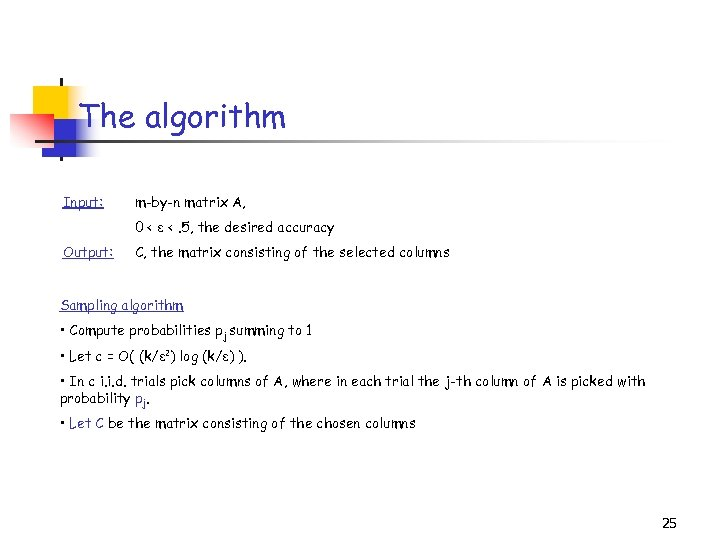The algorithm Input: m-by-n matrix A, 0 < ε <. 5, the desired accuracy Output: C, the matrix consisting of the selected columns Sampling algorithm • Compute probabilities pj summing to 1 • Let c = O( (k/ε 2) log (k/ε) ). • In c i. i. d. trials pick columns of A, where in each trial the j-th column of A is picked with probability pj. • Let C be the matrix consisting of the chosen columns 25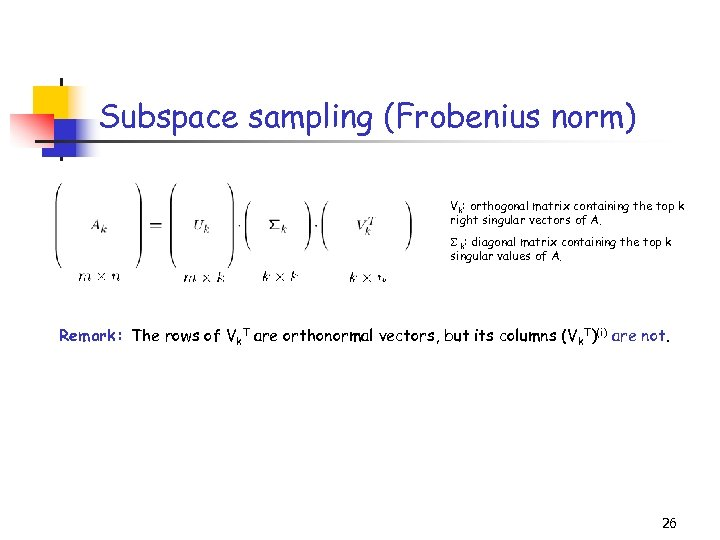Subspace sampling (Frobenius norm) Vk: orthogonal matrix containing the top k right singular vectors of A. S k: diagonal matrix containing the top k singular values of A. Remark: The rows of Vk. T are orthonormal vectors, but its columns (Vk. T)(i) are not. 26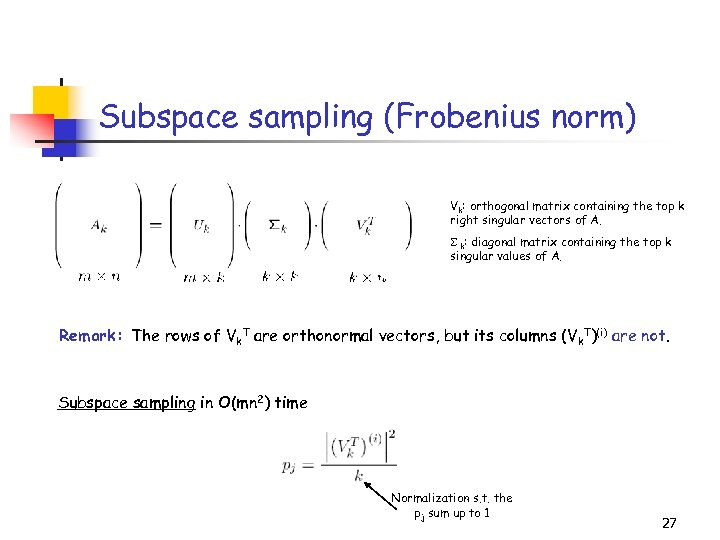Subspace sampling (Frobenius norm) Vk: orthogonal matrix containing the top k right singular vectors of A. S k: diagonal matrix containing the top k singular values of A. Remark: The rows of Vk. T are orthonormal vectors, but its columns (Vk. T)(i) are not. Subspace sampling in O(mn 2) time Normalization s. t. the pj sum up to 1 27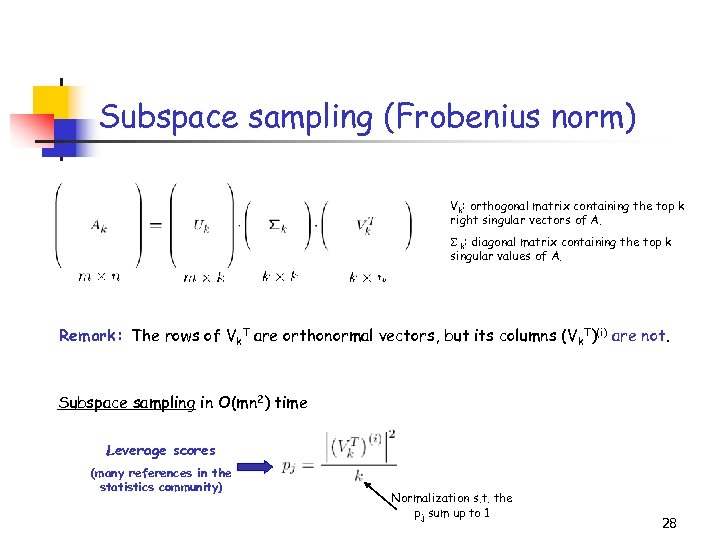Subspace sampling (Frobenius norm) Vk: orthogonal matrix containing the top k right singular vectors of A. S k: diagonal matrix containing the top k singular values of A. Remark: The rows of Vk. T are orthonormal vectors, but its columns (Vk. T)(i) are not. Subspace sampling in O(mn 2) time Leverage scores (many references in the statistics community) Normalization s. t. the pj sum up to 1 28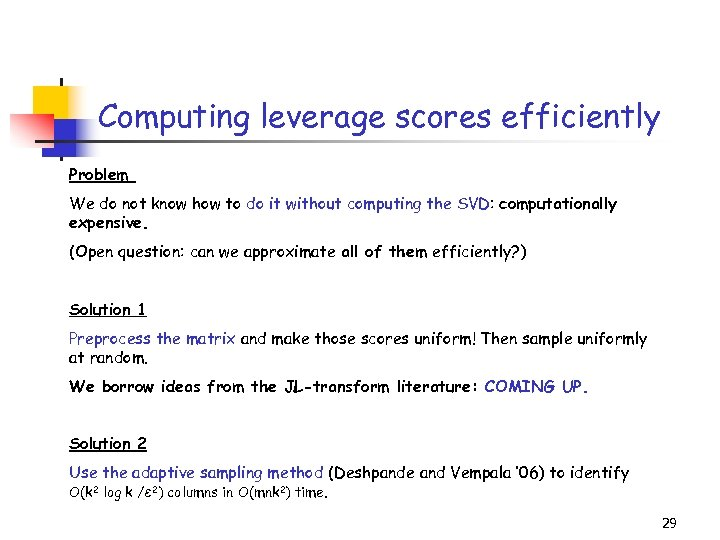Computing leverage scores efficiently Problem We do not know how to do it without computing the SVD: computationally expensive. (Open question: can we approximate all of them efficiently? ) Solution 1 Preprocess the matrix and make those scores uniform! Then sample uniformly at random. We borrow ideas from the JL-transform literature: COMING UP. Solution 2 Use the adaptive sampling method (Deshpande and Vempala ’ 06) to identify O(k 2 log k /ε 2) columns in O(mnk 2) time. 29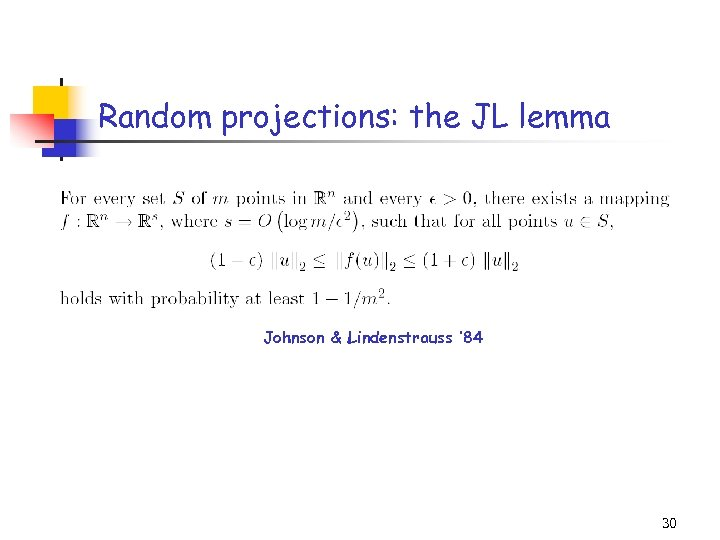Random projections: the JL lemma Johnson & Lindenstrauss ’ 84 30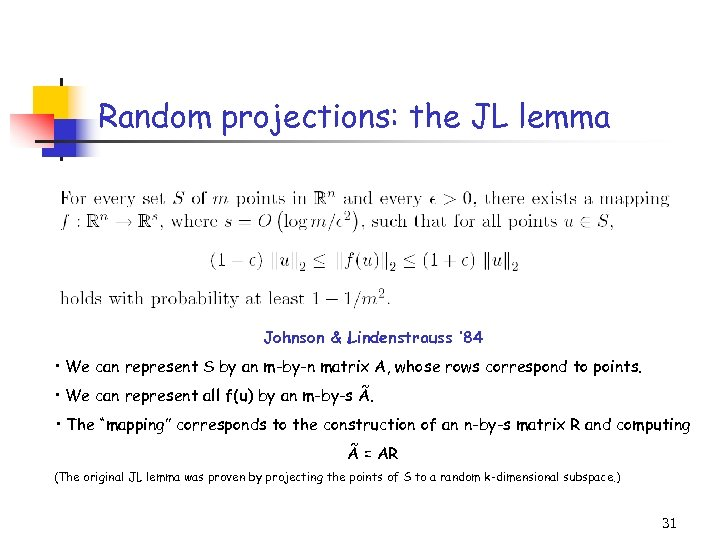Random projections: the JL lemma Johnson & Lindenstrauss ’ 84 • We can represent S by an m-by-n matrix A, whose rows correspond to points. • We can represent all f(u) by an m-by-s Ã. • The “mapping” corresponds to the construction of an n-by-s matrix R and computing Ã = AR (The original JL lemma was proven by projecting the points of S to a random k-dimensional subspace. ) 31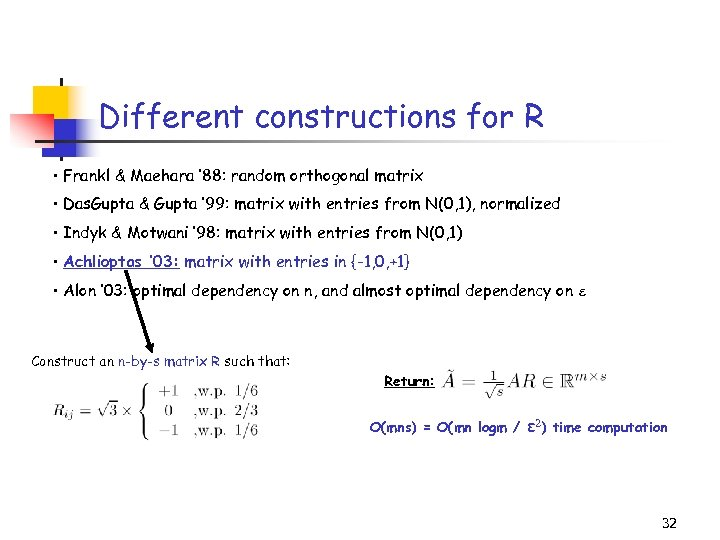Different constructions for R • Frankl & Maehara ’ 88: random orthogonal matrix • Das. Gupta & Gupta ’ 99: matrix with entries from N(0, 1), normalized • Indyk & Motwani ’ 98: matrix with entries from N(0, 1) • Achlioptas ’ 03: matrix with entries in {-1, 0, +1} • Alon ’ 03: optimal dependency on n, and almost optimal dependency on Construct an n-by-s matrix R such that: Return: O(mns) = O(mn logm / ε 2 ) time computation 32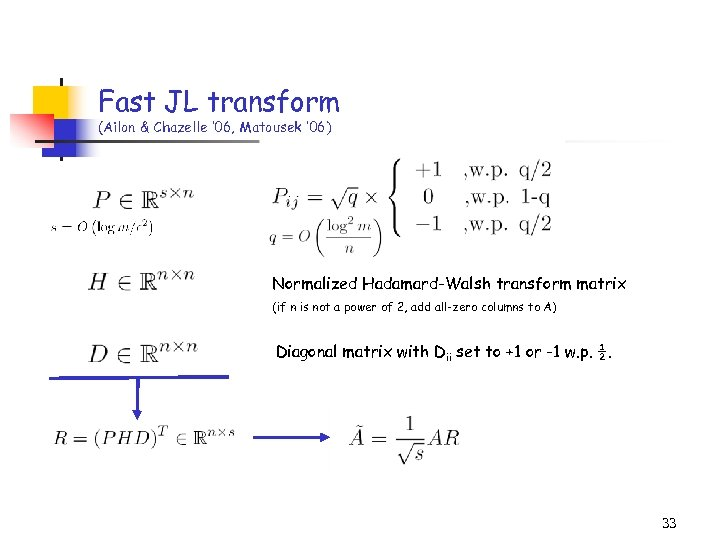Fast JL transform (Ailon & Chazelle ’ 06, Matousek ’ 06) Normalized Hadamard-Walsh transform matrix (if n is not a power of 2, add all-zero columns to A) Diagonal matrix with Dii set to +1 or -1 w. p. ½. 33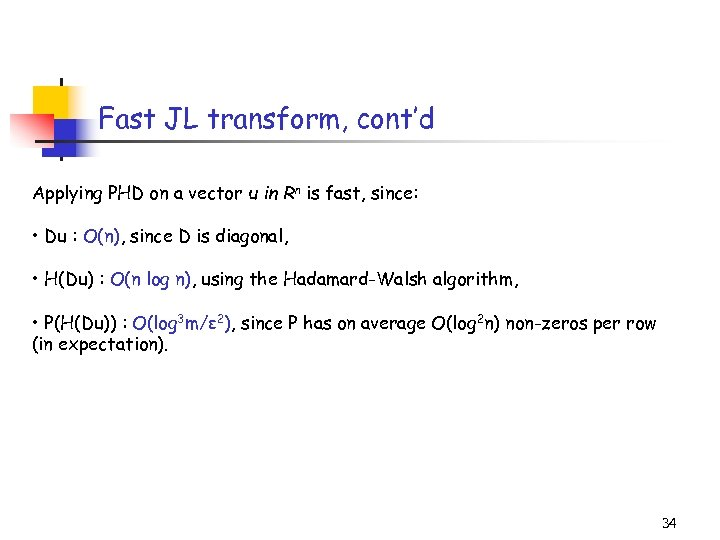Fast JL transform, cont’d Applying PHD on a vector u in Rn is fast, since: • Du : O(n), since D is diagonal, • H(Du) : O(n log n), using the Hadamard-Walsh algorithm, • P(H(Du)) : O(log 3 m/ε 2), since P has on average O(log 2 n) non-zeros per row (in expectation). 34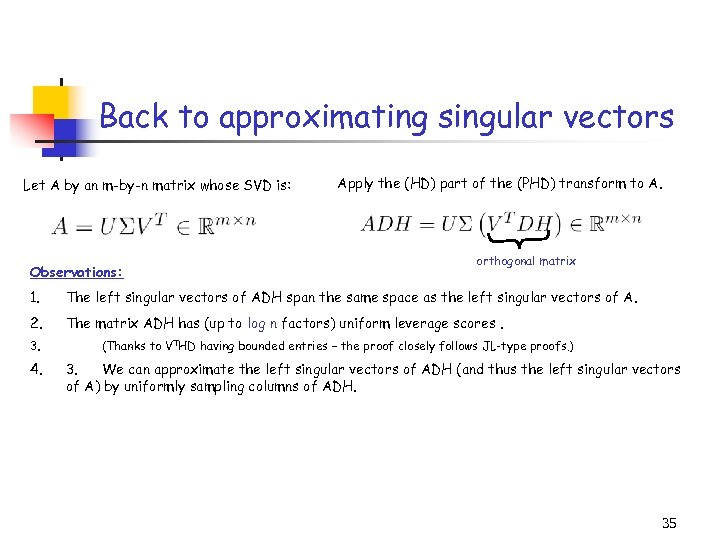Back to approximating singular vectors Let A by an m-by-n matrix whose SVD is: Observations: Apply the (HD) part of the (PHD) transform to A. orthogonal matrix 1. The left singular vectors of ADH span the same space as the left singular vectors of A. 2. The matrix ADH has (up to log n factors) uniform leverage scores. 3. 4. (Thanks to VTHD having bounded entries – the proof closely follows JL-type proofs. ) 3. We can approximate the left singular vectors of ADH (and thus the left singular vectors of A) by uniformly sampling columns of ADH. 35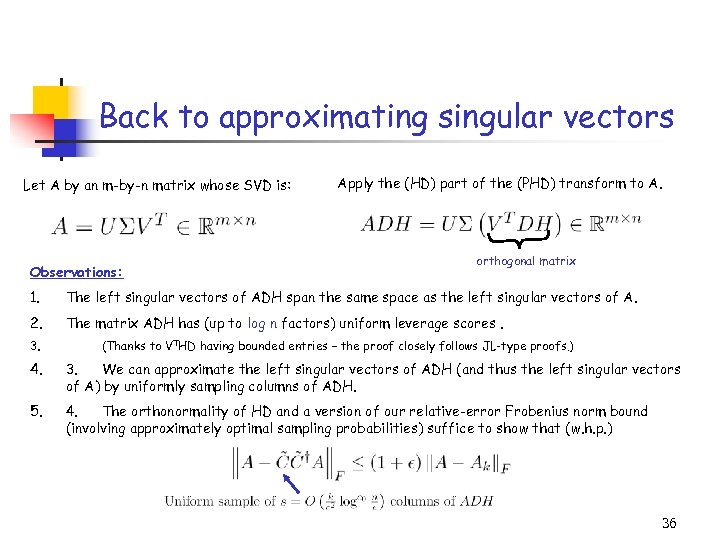Back to approximating singular vectors Let A by an m-by-n matrix whose SVD is: Observations: Apply the (HD) part of the (PHD) transform to A. orthogonal matrix 1. The left singular vectors of ADH span the same space as the left singular vectors of A. 2. The matrix ADH has (up to log n factors) uniform leverage scores. 3. (Thanks to VTHD having bounded entries – the proof closely follows JL-type proofs. ) 4. 3. We can approximate the left singular vectors of ADH (and thus the left singular vectors of A) by uniformly sampling columns of ADH. 5. 4. The orthonormality of HD and a version of our relative-error Frobenius norm bound (involving approximately optimal sampling probabilities) suffice to show that (w. h. p. ) 36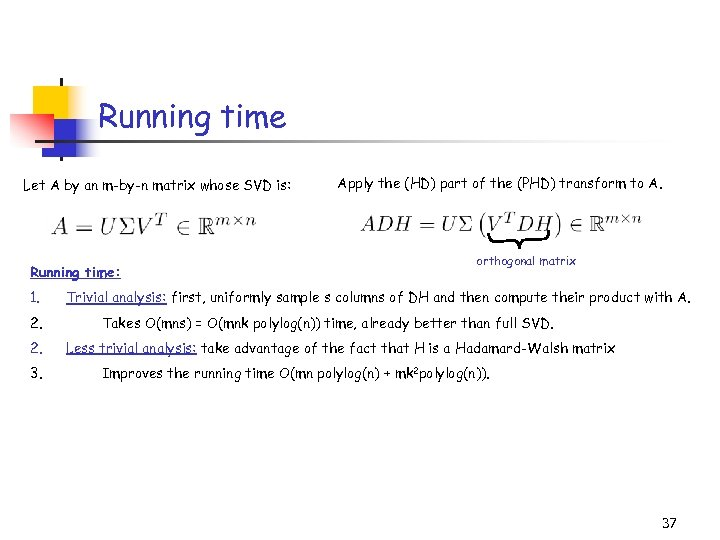Running time Let A by an m-by-n matrix whose SVD is: Running time: 1. 2. 2. 3. Apply the (HD) part of the (PHD) transform to A. orthogonal matrix Trivial analysis: first, uniformly sample s columns of DH and then compute their product with A. Takes O(mns) = O(mnk polylog(n)) time, already better than full SVD. Less trivial analysis: take advantage of the fact that H is a Hadamard-Walsh matrix Improves the running time O(mn polylog(n) + mk 2 polylog(n)). 37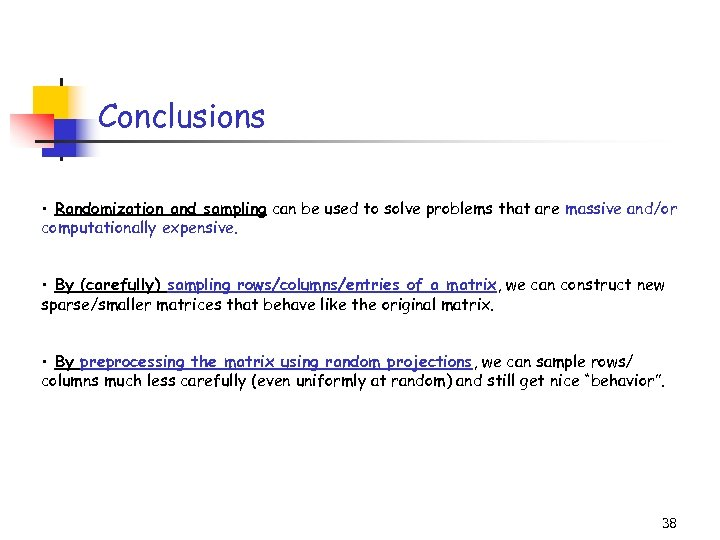Conclusions • Randomization and sampling can be used to solve problems that are massive and/or computationally expensive. • By (carefully) sampling rows/columns/entries of a matrix, we can construct new sparse/smaller matrices that behave like the original matrix. • By preprocessing the matrix using random projections, we can sample rows/ columns much less carefully (even uniformly at random) and still get nice “behavior”. 38